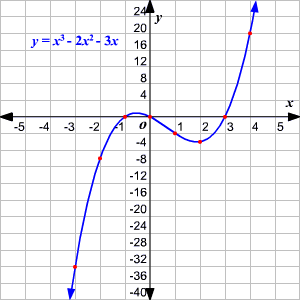# Graphing Polynomial Functions

To sketch any polynomial function, you can start by finding the real zeros of the function and end behavior of the function .

Steps involved in graphing polynomial functions:

$1$ . Predict the end behavior of the function.

$2$ . Find the real zeros of the function. Check whether it is possible to rewrite the function in factored form to find the zeros. Otherwise, use Descartes' rule of signs to identify the possible number of real zeros.

$3$ . Make a table of values to find several points.

$4$ . Plot the points and draw a smooth continuous curve to connect the points.

$5$ . Make sure that the graph follows the end behavior as found in the above step.

Example:

Graph the polynomial function ${x}^{3}-2{x}^{2}-3x$ .

Predict the end behavior of the function.

The degree of the polynomial function is odd and the leading coefficient is positive.

The degree of the polynomial is $3$ and there would be $3$ zeros for the functions.

The function can be factored as $x\left(x+1\right)\left(x-3\right)$ . So, the zeros of the functions are $x=-1,0$ and $3$ .

Make a table of values to find several points.

 $x$ $f\left(x\right)$ $-3$ $-36$ $-2$ $-10$ $-1$ $0$ $0$ $0$ $1$ $-4$ $2$ $-6$ $3$ $0$ $4$ $20$

Plot the points and draw a smooth continuous curve to connect the points